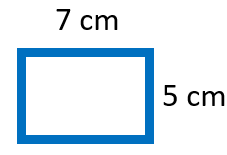# Math Worksheets Land

Math Worksheets For All Ages

# Math Worksheets Land

Math Worksheets For All Ages

# Simple Area of a Rectangle Worksheets

Rectangles are one of the most fundamental shapes that we begin to work with when we first approach geometry. This is one of the most common shapes you will find in building. Almost all rooms are found in this shape. Rectangles have 4 unequal adjacent sides. The opposite sides are equal sizes. This leaves a rectangle with two measures length (longest sides) and width (smallest sides). The two-dimensional space that a rectangle occupies is referred to as area. This measure, no matter how simple it may be to calculate, is critical to many areas of international trade. These worksheets and lessons will help you learn how to determine the area of rectangles quickly and efficiently. We have a full walkthrough in our lessons here and you will also find a solution to example problem at the bottom of this page.

### Aligned Standard: Grade 3 Measurement - 3.MD.7

• Answer Keys - These are for all the unlocked materials above.

### Homework Sheets

We start off with basic problems and then give them purpose by adding the fact that they are rooms.

• Homework 1- Find the area of the rectangles. All measures are in meters. It might help to add more labels.
• Homework 2- A rectangular room measures 9 m by 4 m. A typical problem you will see here.
• Homework 3- We continue on with this skill. A rectangular room measures 17 m by 10 m.

### Practice Worksheets

We transition from rooms to outdoor fields.

• Practice 1-A rectangular field measures 21 m by 17 m. What can you tell us about this field?
• Practice 2- See if you can make sense of what is going on here.
• Practice 3- A rectangular hall measures 15 m by 10 m. What is cool about this hall?

### Math Skill Quizzes

By the end of this area students should have a solid understanding of square footage.

• Quiz 1- A rectangular room measures 5 m by 4 m. That is a pretty big room.
• Quiz 2- A rectangular room measures 7 m by 6 m. Aren't most rooms that shape?
• Quiz 3- More about the rooms. It is like you are an architect or something.

### How to Determine Simple Area of a Rectangle

A rectangle is a 2-dimensional shape with four sides. The opposite sides of the rectangle are equal, and the angle between the two sides is 90° . Every two-dimensional shape covers an area and has a perimeter. These shapes do not have a volume or a surface area.

Measuring the area of a rectangle is simple. All you must do is identify the length of a rectangle and its width. The length is the longest side and the width is one of the sides that is not parallel to the length. The product of these two quantities will give you the total area of the rectangle.

The formula to calculate the area of the rectangle is; A = L x W

A = Area, L = Length, W = Width

Here is a representation that will help you identify the length and width of a rectangle.

Example Problem: Find the area of the following shape.The length (longest side) here is 7 cm. The width (non-parallel side to length) here is 5 cm.

Using the formula: Area = Length x Width

A = 7 cm x 5 cm = 35 cm2. The area of this rectangle is 35 square centimeters.

### Why is This Measure Important?

The measure of area two us the extent of figure we are examining in two dimensions. This gives us a general understanding of how big a room is, but not the full picture because we are not examining height of the ceiling. This skill leads us to be able to determine the square footage of an area. In the United States this measure is critical to many different industries mainly construction. Home builders base their price for building a home entire on how many square feet the home will be. An average home will cost \$100-\$200 per square foot to build. For a luxury home built with more expensive materials that can reach \$300-\$500 per square foot. When you have work done on your home such as decking, tiling, roofing, or flooring contractors will charge based on this measure. The quality of materials used will change this price. When purchasing home real estate investors use this measure to determine if they are getting a deal or paying too much for their investment. While this may seem like a redundant topic for you to explore, I can assure you big business is obsessed with this measure.

Unlock all the answers, worksheets, homework, tests and more!
Save Tons of Time! Make My Life Easier Now

## Thanks and Don't Forget To Tell Your Friends!

I would appreciate everyone letting me know if you find any errors. I'm getting a little older these days and my eyes are going. Please contact me, to let me know. I'll fix it ASAP.